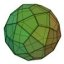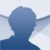Limix Geometric

1.3.25

Download Limix Geometric for free, a geometrical calculation tool. With it you can find out the area and surface of all kinds of geometrical shapes

Mathematical and geometrical calculations are usually complicated matters, especially at beginner levels. The formulas that are used are relatively complicated and not always easy to remember, that is why Limix Geometric can help us in more than one situation, especially when it comes to studying this part of maths.

Carry out geometric operations on your PC

Thanks to Limix Geometric, obtaining the area and the surface of the most usual geometric figures won't be difficult at all, because it has practically any of them ready to be calculated by simply imputing the data that the program will ask for.

The figures that it can calculate are: squares, rectangles, rhombus, triangles, trapezoids, polygons, circles, a circular sector, ellipse, cubes, prisms, pyramids, cylinders, cones, body of a cone, tetrahedrons, octahedron, dodecahedron, icosahedron, spheres,...

All the calculations show the formulas that are used and a diagram that explains the calculation, something that can come in very handy for students that have problems learning geometry and its formulas.

Limix Software
Over a year ago
More than a year ago
82 KB

We'd love to hear from you. Do you want to give us your opinion?Logged off